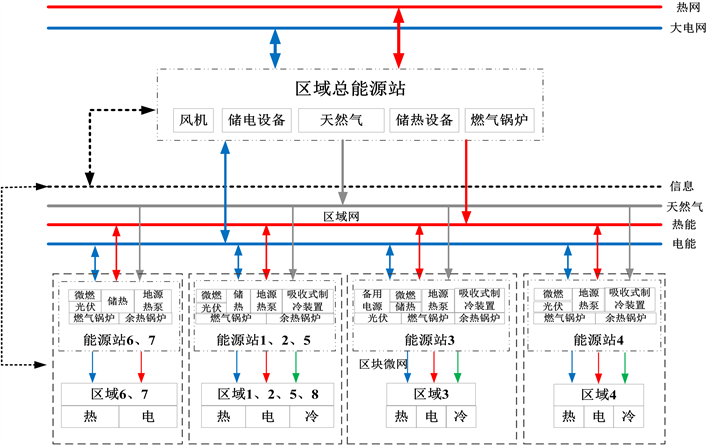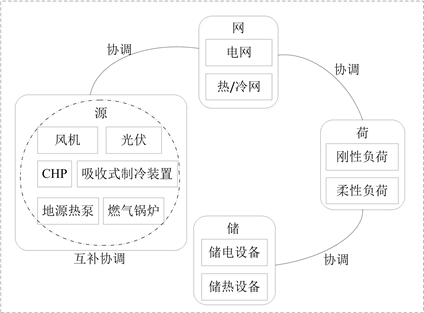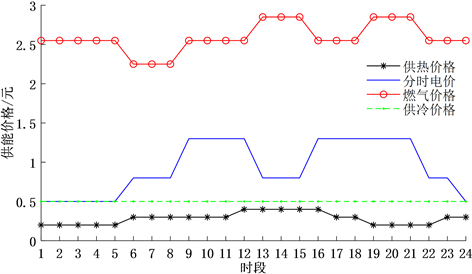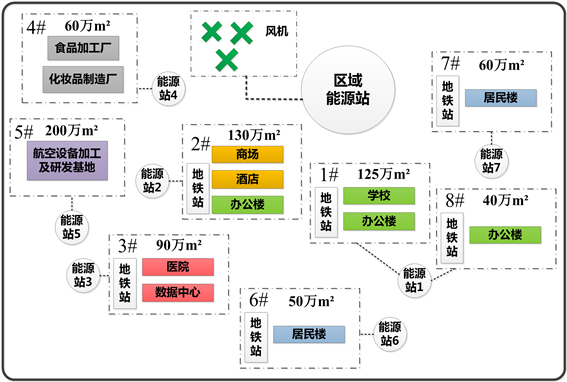# 基于能源互联网的多能源系统综合优化规划Comprehensive Optimization Programming of Multi-Energy System Based on Energy Internet

• 全文下载: PDF(1410KB)    PP.571-579   DOI: 10.12677/SG.2018.86063
• 下载量: 218  浏览量: 412

Based on the concept of energy Internet, the multi-energy system optimization programming considering complementarity and sharing of electricity, heat and cold is the basis to improve the multi-energy utilization. The multi-energy system is supplied by multiple distributed energy stations, which are connected by the power supply network and heating network on the basis of self-balancing capability. The multi-source complementarity is realized, and the “generation-grid-load-storage” coordination strategy is put forward. Considering the sizing cost and the typical scenario operation revenue, carbon emissions and primary energy consumption, and the feasibility and security constraints, multi-energy system optimal programming mathematical model is established. Analysis shows that the multi-energy system programming scheme which is considering the typical scenarios operation achieves the optimal effect of sizing cost and running comprehensive benefit.

1. 引言

2. 多能源系统互补协调

2.1. 横向多源互补简介Figure 1. Multi-energy system construction

2.2. 纵向“源–网–荷–储”协调策略Figure 2. Technology roadmap of double trace

3. 多能源系统优化规划数学模型

$\mathrm{max}F=-{f}_{1}+{f}_{2}$ (1)

s.t.

${P}_{CHP.e.t}+{P}_{grid.e.t}+{P}_{new.t}={L}_{e.t}-{L}_{cut.e.t}+{P}_{BT.e.t}+{P}_{HP.e.t}$ (2)

${P}_{CHP.h.t}+{P}_{GB.h.t}+{P}_{HP.h.t}+{P}_{grid.h.t}={L}_{h.t}-{L}_{cut.h.t}+{P}_{BT.h.t}+{P}_{AC.h.t}$ (3)

${P}_{AC.c.t}={L}_{c.t}$ (4)

$0\le {P}_{j.grid.e.t}\le {P}_{j.grid.e.\mathrm{max}}$ (5)

$0\le {P}_{j.grid.h.t}\le {P}_{j.grid.h.\mathrm{max}}$ (6)

$\underset{i=1}{\overset{{n}_{1}}{\sum }}{P}_{i.e.\mathrm{max}}+{P}_{grid.e.\mathrm{max}}>\mathrm{max}\left({L}_{e}-{P}_{new}\right)\left(1+10%\right)$ (7)

$\underset{i=1}{\overset{{n}_{2}}{\sum }}{P}_{i.h.\mathrm{max}}+{P}_{grid.h.\mathrm{max}}>{L}_{h.\mathrm{max}}\left(1+10%\right)$ (8)

$\mathrm{min}{f}_{1}=\underset{j=1}{\overset{8}{\sum }}\underset{i=1}{\overset{N}{\sum }}{C}_{i}×{P}_{j.i.\mathrm{max}}\frac{r{\left(1+r\right)}^{{y}_{i}}}{{\left(1+r\right)}^{{y}_{i}}-1}$ (9)

$\mathrm{max}{f}_{2}={g}_{1}-{\beta }_{2}{g}_{2}-{\beta }_{3}{g}_{3}$ (10)

${g}_{1}=\underset{t=1}{\overset{24}{\sum }}\left[{C}_{sale.t}-{C}_{buy.t}-{C}_{OM.t}\right]$ (11)

${g}_{2}=\underset{t=1}{\overset{24}{\sum }}\left[{Q}_{gas.t}×{\mu }_{gas}+{P}_{grid.e.t}×{\mu }_{e}\right]$ (12)

${g}_{3}=\underset{t=1}{\overset{24}{\sum }}{Q}_{gas.t}×{\sigma }_{gas}+{P}_{grid.e.t}×{\sigma }_{e}$ (13)

${C}_{sale.t}={C}_{e.t}×{L}_{e.t}+{C}_{h.t}×{L}_{h.t}+{C}_{c.t}×{L}_{c.t}$ (14)

${C}_{buy.t}=\frac{{Q}_{gas.t}}{LH{V}_{n}}×{C}_{gas.t}\left({Q}_{gas.t}\right)+{P}_{grid.e.t}×{C}_{e.t}+{P}_{grid.h.t}×{C}_{h.t}$ (15)

${Q}_{gas.t}=\frac{{P}_{CHP.e.t}}{{\eta }_{CHP}}+\frac{{P}_{GB.h.t}}{{\eta }_{GB}}$ (16)

${C}_{gas.t}\left({Q}_{gas.t}\right)=\left\{\begin{array}{l}{C}_{n.p}\text{\hspace{0.17em}}\text{\hspace{0.17em}}\text{\hspace{0.17em}}\text{\hspace{0.17em}}{Q}_{gas.t}\in \left[{Q}_{p.\mathrm{min}},{Q}_{p.\mathrm{max}}\right]\\ {C}_{n.f}\text{\hspace{0.17em}}\text{\hspace{0.17em}}\text{\hspace{0.17em}}\text{\hspace{0.17em}}{Q}_{gas.t}\in \left[{Q}_{f.\mathrm{min}},{Q}_{f.\mathrm{max}}\right]\\ {C}_{n.v}\text{\hspace{0.17em}}\text{\hspace{0.17em}}\text{\hspace{0.17em}}\text{\hspace{0.17em}}{Q}_{gas.t}\in \left[{Q}_{v.\mathrm{min}},{Q}_{v.\mathrm{max}}\right]\end{array}$ (17)

${C}_{OM.t}=\underset{i=1}{\overset{N}{\sum }}{P}_{i.t}×{C}_{OM.i}$ (18)

4. 算例分析Table 1. Energy consumption characteristics of buildingsTable 2. Daily cooling heating and electrical load of buildingsTable 3. Parameters of distributed energy resourceFigure 3. Time-of-use energy price

4.1. 基于区块划分的能源站分布Figure 4. Programme of area blocks

4.2. 兼顾运行的多能源系统优化规划结果分析Table 4. Optimal sizing result of the energy stationsTable 5. The indexes comparisons between different optimal sizing methods

5. 结论

1) 兼顾典型场景运行效益的多能源系统规划方案，年净收益增加的幅值远大于年等值投资成本，缩短了多源系统投资回收年限。

2) 在规划方案中考虑了系统运行的碳排放和一次能源消耗量，大幅度降低了碳排放量和一次能源消耗量，提高了多能系统的环保性和节能性。

3) 在需求侧响应的基础上，通过“源–网–荷–储”协调策略，跟踪风机和光伏出力，平滑了负荷曲线，降低了多能源系统购能成本。

  Rifkin, J. (2011) The Third Industrial Revolution: How Lateral Power Is Transforming Energy, Economy, and the World. Palgrave MacMillan, New York, 46-48.  Stanislav, P., Bryan, K. and Tihomir, M. (2009) Smart Grids Better with Integrated Energy System. 2009 IEEE Electrical Power & Energy Conference (EPEC), Montreal, QC, 22-23 October 2009, 1-8.  曾鸣. 我国向清洁能源转型及其实现过程中的两个关键问题[N]. 国家电网报, 2014-09-26(1).  曾鸣. 混合能源背景下的电力规划[N]. 上海证券报, 2014-10-16(A1).  曾鸣, 杨雍琦, 武赓, 等. “源–网–荷–储”协调优化是构建能源互联网的关键[Z]. 电力系统自动化微文, 2015.  曾鸣, 杨雍琦, 李源非, 等. 能源互联网背景下新能源电力系统运营模式及关键技术初探[J]. 中国电机工程学报, 2016, 36(3) :681-691.  Masood, E. and Ali, K. (2013) Sizing the Prime Mover of a Residential Micro-Combined Cooling Heating and Power (CCHP) System by Multi-Criteria Sizing Method for Different Climates. Energy, 54, 291-301.  肖小清, 阚伟民, 杨允, 等. 有蓄能的联供系统超结构优化配置[J]. 中国电机工程学报, 2012, 32(32): 8-14.  吴利辉, 杨洪海, 吴植华. 三联供系统配置及运行策略的多目标优化分析[J]. 建筑热能通风调, 2012, 31(1): 16-18.  荆有印, 白鹤, 张建良. 太阳能冷热电联供系统的多目标优化设计与运行策略分析[J]. 中国电机工程学报, 2012, 32(20): 82-87.  Yokoyama, R. and Ito, K. (2004) Optimal Design of Gas Turbine Cogeneration Plants in Consideration of Discreteness of Equipment. Capacities. Proceed-ings of ASME Turbo Expo 2004: Power for Land, Sea, and Air, Vienna, 14-17 June 2004.  甘霖, 陈瑜玮, 刘育权, 等. 含可再生能源的微网冷–热–电多能流协同优化与案例分析[J]. 电力自动化设备, 2017, 37(6): 275-281.  赵峰, 张承慧, 孙波, 等. 冷热电联供系统的三级协同整体优化设计方法[J]. 中国电机工程学报, 2015, 35(15): 3785-3793.  段绍辉, 汪伟, 刘中胜, 等. 含光伏的冷热电联供微网系统优化调度方案[J]. 电力系统及其自动化学报, 2013, 25(4): 150-155.  王艳艳. 基于可再生能源的城区建筑能源体系规划研究[D]: [硕士学位论文]. 兰州: 兰州交通大学, 2015.